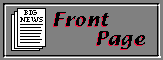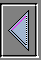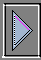# Exploring parsing and virtual machines with Python

#### By Pramode C E

The design of compilers/interpreters is a challenging field - one which offers a lot of scope for theoretical exploration as well as hands on coding. Being a Python fan, I tried to implement some of the ideas which I am learning about compilers/interpreters in this beautiful language. As I am neither a Python Guru nor a compiler expert, the implementation may be imperfect. But it was certainly lots of fun!

## A simple language

Don't be disappointed when I tell you that we are not going to discuss the implementation of an Object Oriented, functional language with automatic garbage collection and the works! The language I am talking about here is the one which we learn as kids, the language of arithmetic expressions. For example,
```
1+2*3-4
1/2+3-4/5
.....
```
We will start with a program which will read an expression of this form and evaluate it directly. We will then modify this program to generate a data structure called a parse tree which can then be evaluated by recursive algorithms. The next step is to generate instructions for a virtual machine using this parse tree. The last step is to store these virtual machine instructions on disk and run it with an interpreter when required.

## Context-free grammars

Programming languages are often described using a compact and powerful notation called a Context-free Grammar. The grammar describes a set of substitutions. Here is a grammar for arithmetic expressions:

```
E ::= T { ADDOP T }
T ::= F { MULOP F }
F ::= 0 | 1 | 2 | 3 | .....
MULOP ::= * | /

```
Assume that E stands for expression, T stands for term and F stands for factor. The curly brace denotes 'zero or more repetitions'. Reading the first production, we would say that "An expression is a term, followed by zero or more repetitions of the combination of an adding operator and a term." The third production says that a factor is either 0 or 1 or 2 or 3 or 4 and so on, ie, the whole set of positive integers. It takes some time to get used to esoteric definitions like this, but if we have a basic understanding of recursive structures, it is not very difficult.

## A simple expression evaluator

Here is the source for a simple expression evaluator in Python. (text version)

```
#--------------------A simple expression evaluator---------------#

import re, string
Inputbuf = []

# A token is either a number or an operator symbol.
# The main program reads a line from the input and
# stores it in an array called Inputbuf. The function
# gettoken() returns individual tokens from this array.

def gettoken():
global Inputbuf
p = re.search('^\W*[\+\-\*/]|^\W*[0-9]+', Inputbuf)
token = p.string[p.regs:p.regs]
token = string.strip(token)
if token not in ['+', '-', '*', '/']:
token = int(token)
Inputbuf = Inputbuf[p.regs:]

# lookahead() peeks into the input stream and tells you what
# the next input token is

global Inputbuf
try:
p = re.search('^\W*[\+\-\*/]|^\W*[0-9]+', Inputbuf)
token = p.string[p.regs:p.regs]
token = string.strip(token)
if token not in ['+', '-', '*', '/']:
token = int(token)
except:
return None

def factor():
return gettoken()

def term():
e1 = factor()
while (tmp in ['*', '/']):
gettoken()
if (tmp == '*'):
e1 = e1 * factor()
else:
e1 = e1 / factor()

return e1

def expression():
e1 = term()
while (tmp in ['+', '-']):
gettoken()
if (tmp == '+'):
e1 = e1 + term()
else:
e1 = e1 - term()

return e1

def main():
global Inputbuf
Inputbuf = raw_input()
print expression()

if __name__=='__main__':
main()

```
It would be good to trace the execution of the above code for some simple expressions.

## Producing a parse tree

The above program simply evaluates the given infix arithmetic expression. We are now going to modify it to produce a parse tree instead. A parse tree for the expression 1+2*3 would look like this:

```			+
/ \
/   \
1     *
/ \
/   \
2   3
```
Each node of the tree consists of the following fields:
1. 'op' or 'number' depending on whether the node is an internal node or a leaf node
2. A link to the 'left child' called 'left'
3. A link to the 'right child' called 'right'
The tree is built from the bottom up. The function 'factor' simply creates a new tree node with a number in it, initializes the left and right pointers to NULL, and returns the node. The function 'expression()' creates a new node with an operator '+' or '-' as the value of the 'op' field and assigns the left and right pointers to values obtained by calling 'term()'. Function 'term()' works in a similar way.
```
#--------------------Produce a parse tree---------------------#

# gettoken() and lookahead() are same as in the first listing

NULL = 0
import re, string
Inputbuf = []

class Tree:
pass

def factor():
newnode = Tree()
newnode.number = gettoken()
newnode.left = newnode.right = 0
return newnode

def term():
left = factor()
while (tmp in ['*', '/']):
gettoken()
right = factor()
newnode = Tree()
newnode.op = tmp
newnode.left = left
newnode.right = right
left = newnode

return left

def expression():
left = term()
while (tmp in ['+', '-']):
gettoken()
right = term()
newnode = Tree()
newnode.op = tmp
newnode.left = left
newnode.right = right
left = newnode

return left

def treeprint(ptree):
if (ptree):
try:
print ptree.op
except:
print ptree.number
treeprint(ptree.left)
treeprint(ptree.right)

def main():
global Inputbuf
Inputbuf = raw_input()
ptree = expression()
return ptree

if __name__=='__main__':
ptree = main()
treeprint(ptree)
```

## Building a stack machine

The parse tree which we have created can be easily evaluated by writing a recursive function. But we will adopt a different method. We will generate code for evaluating expressions in the instruction set of a simple hypothetical machine called a 'stack machine'. The instructions which this machine has are very simple - push a number on to the stack, add two numbers, multiply two numbers etc. Thus, evaluation of the expression 1+2*3 yields the following code:

```push 1
push 2
push 3
mul
```
These instructions are stored in an array. Push, mul, add etc are functions. The instructions may be directly executed by walking through the array and executing the functions held by each array element or they may stored in a disk file (an easy way is to use the Python pickle module, though it is a waste of space). Another program may then read this code into an array and execute it. The code which I have written works like this: If you run the program without any filename argument, it reads an expression from the keyboard, generates code for the virtual machine in an array and executes it by walking through the array. The code is also stored in a file called 'code.out'. Now if you run the program with a file name argument code.out, it loads the instructions from the file and executes it, without reading from the keyboard.
```
import re, string, sys, pickle
# Functions not included herein should be copied from the previous listings.

NULL = 0
Inputbuf = []

NCODE = 100
NSTACK = 100
Code = []
Stack =  * NSTACK
Pc = 0
Stackp = 0

class Tree:
pass

class CodeItem:
pass

def initcode():
global Code
for i in range(0, NCODE):
t = CodeItem()
Code.append(t)

def pushop():
global Stack, Stackp, Code, Pc

Stack[Stackp] = Code[Pc].number
Stackp = Stackp + 1
Pc = Pc + 1

global Stack, Stackp, Code, Pc

Stackp = Stackp - 1
right = Stack[Stackp]
Stackp = Stackp - 1
left = Stack[Stackp]
Stack[Stackp] = left + right
Stackp = Stackp + 1

# define subop, mulop and divop here.

def generate(codep, ptree):
try:
# if the field 'number' is not present, the
# following line generates an exception.

n = ptree.number
Code[codep].op = pushop
codep = codep + 1
Code[codep].number = n
codep = codep + 1
return codep
except:
if (ptree.op == '+'):
codep = generate(codep, ptree.left)
codep = generate(codep, ptree.right)
codep = codep + 1
return codep

# elif (ptree.op == '-'): We will write the code
# generation actions for '-', '*', '/' here.

def eval(ptree): # Generate the instructions, then execute them
global Pc, Stackp, Code, Stack
Pc = generate(0, ptree)
Code[Pc].op = NULL

Stackp = 0
Pc = 0
while Code[Pc].op != NULL:
tmp = Pc
Pc = Pc + 1
Code[tmp].op()
return Stack

def eval2():    # Directly execute the loaded code
global Pc, Stackp, Code, Stack

Stackp = 0
Pc = 0
while Code[Pc].op != NULL:
tmp = Pc
Pc = Pc + 1
Code[tmp].op()
return Stack

def main():
global Inputbuf, Code

try:
f = open(sys.argv)
f.close()
result = eval2()
print 'result is:', result
return result
except:
print 'Not opening code file, reading from k/b'
initcode()
Inputbuf = raw_input()
ptree = expression()
result = eval(ptree)
f = open('code.out', 'w')
pickle.dump(Code, f)
print 'Code dumped in a file called dat'
print 'result is:', result
return result

if __name__=='__main__':
result = main()

```
'generate()' and 'eval()' are the critical functions. 'generate()' walks through the expression tree creating the virtual machine code and storing it in an array 'Code'. 'eval()' walks through the array 'Code' executing the instructions, using an array 'Stack' for holding the partial results.

## Conclusion

It is possible to extend the above program to handle variables and assignment statements, control flow constructs like gotos, if statements etc. Soon, you would be building a simple Basic-like language.

Coming from a C background, Python's lack of certain C constructs like the ++ operator is a minor irritation. The lack of compile time type declarations also seems to have some detrimental effects upon code readability. Also, you will pay dearly for any typo. If you have a variable 'f' of type 'struct foo' and 'foo' does not have a field called 'next', an assignment to 'f.next' will generate a compile time error in C whereas the Python interpreter would gladly allow the assignment to go through.

## References

The standard book on compiler design is 'Principles of Compiler Design' by Aho A.V and Ullman J.D. The inspiration for this article came from 'The Practice of Programming', another excellent book by Brian Kernighan and Rob Pike. The 'generate' and 'eval' functions are Python renderings of code from this book. 'A Second course in Computer Science with Pascal' by Daniel D. McCracken presents several algorithms, including an expression evaluator, in a very engaging style.